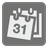Days Between
Elementary
JA English EL FR IT RU UK ZH-CN
We have prepared a set of Editor's Choice Solutions. You will see them first after you solve the mission. In order to see all other solutions you should change the filter.

How old are you in a number of days? It's easy to calculate - just subtract your birthday from today. We could make this a real challenge though and count the difference between any dates.

You are given two dates as an array with three numbers - a year, month and day. For example: 19 April 1982 will be (1982, 4, 19). You should find the difference in days between the given dates. For example between today and tomorrow = 1 day. The difference will always be either a positive number or zero, so don't forget about the absolute value.

Input: Two dates as tuples of integers.

Output: The difference between the dates in days as an integer.

Example:

```days_diff((1982, 4, 19), (1982, 4, 22)) == 3
days_diff((2014, 1, 1), (2014, 8, 27)) == 238
```

How it is used: Python has batteries included, so in this mission you’ll need to learn how to use completed modules so that you don't have to invent the bicycle all over again.

Precondition: Dates between 1 january 1 and 31 december 9999. Dates are correct.